# RD Sharma Solutions Class 9 Chapter 4 Algebraic Identities

Read RD Sharma Solutions Class 9 Chapter 4 Algebraic Identities below, students should study RD Sharma class 9 Mathematics available on Studiestoday.com with solved questions and answers. These chapter wise answers for class 9 Mathematics have been prepared by teacher of Grade 9. These RD Sharma class 9 Solutions have been designed as per the latest NCERT syllabus for class 9 and if practiced thoroughly can help you to score good marks in standard 9 Mathematics class tests and examinations

Exercise 4.1
Question 1: Evaluate each of the following using identities:
(i) (2x – 1/x)2
(ii) (2x+y) (2x – y)
(iii) (a2 b-b2a)2
(iv) (a – 0.1) (a + 0.1)
(v) (1.5.x2 – 0.3y2) (1.5x2 + 0.3y2)
Solution: 1
(i) (2x – 1/x)2
By using identity of (a – b)2 = a2 + b2 – 2ab
(2x –  1/x )2
By using identity we get,
(2x)2 + (1/x)2 – 2 (2x) 1/x
4x2  +  1/x2  – 4

(ii) (2x + y) (2x – y)
By using identity of (a – b)2 = a2 + b2 – 2ab
(2x + y) (2x – y)
By using identity we get,
(2x)2 –(y)2
4x2  – y2

(iii) (a2 b – b2 a)2
By using identity of (a – b)2 = a2 + b2 – 2ab
(a2 b –b2 a)2
By using identity we get,
(a2 b)2+(b2 a)2 – 2 (a2 b)(b2 a)
a4 b2+b4 a2  –2a3 b3

(iv) (a –0.1) (a+0.1)
By using identity of (a – b)2 = a2 + b2 – 2ab
(a –0.1) (a+0.1)
By using identity we get,
(a)2  – (0.1)2
(a)2  –0.01

(v) (1.5.x2 – 0.3y2) (1.5x2 + 0.3y2)
By using identity of (a – b)2 = a2 + b2 – 2ab
(1.5.x2 – 0.3y2) (1.5x2 + 0.3y2)
By using identity we get,
(1.5 x2 )2  – (0.3y2 )2
2.25 x4  – 0.09y4

Question 2: Evaluate each of the following using identities:
(i) (399)2
(ii) (0.98)2
(iii) 991 x 1009
(iv) 117 x 83
Solution: 2
(i) It is given that,
(399)2=(400-1)2
(400)2-(1)2-2×400×1
By using identity of (a-b)2=a2+b2-2ab
a = 400
b = 1
(400)2+(1)2-2×400 ×1
160000 + 1 – 800
159201
Hence, the value of (399)2 is 159201

(ii) It is given that,
(0.98)2=(1-0.02)2
(1)2+(0.02)2-2×1×0.02
By using identity of (a-b)2=a2+b2-2ab
1+0.0004-0.04
1.0004-0.04
0.9604
So, (0.98)2 is 0.9604

(iii) It is given that,
991×1009
(1000-9)  (1000+9)
By using identity of (a-b)2=a2+b2-2ab
( 1000)2-(9)2
1000000 – 81
999919
So, 991×1009 is 99999

(iv) It is given that,
117 × 83
(100+17)(100-17)
By using identity of (a-b)2=a2+b2-2ab
(100)2-(17)2
10000 – 289
9711
Hence, 117×83 is 9711

Question 3: Simplify each of the following:
(i) 175 x 175 +2 x 175 x 25 + 25 x 25
(ii) 322 x 322 – 2 x 322 x 22 + 22 x 22
(iii) 0.76 x 0.76 + 2 x 0.76 x 0.24 + 0.24 x 0.24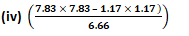Solution: 3
(i) 175 x 175 +2 x 175 x 25 + 25 x 25
= (175)2+2 (175) (25)+(25)2
By the using identity a2+b2+2ab=(a+b)2
= (175+25)2
= (200)2
= 40000
Hence, the simplest form of 175 x 175 +2 x 175 x 25 + 25 x 25 is  40000.

(ii) 322 x 322 – 2 x 322 x 22 + 22 x 22
= (322)2 – 2 x 322 x 22 + (22)2
By the using identity a2+b2-2ab=(a-b)2
= (322 – 22)2
= (300)2
= 90000
Hence, the simplest form of 322 x 322 – 2 x 322 x 22 + 22 x 22 is 90000.

(iii) 0.76 x 0.76 + 2 x 0.76 x 0.24 + 0.24 x 0.24
= (0.76) 2 + 2 x 0.76 x 0.24 + (0.24) 2
By the using identity a2+b2+2ab=(a+b)2
= (0.76 + 0.24)2
= (1.00)2
= 1
Hence, the simplest form of 0.76 x 0.76 + 2 x 0.76 x 0.24 + 0.24 x 0.24 is 1.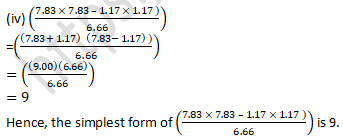Question 4: If x+1/x=11, find the value of x2+1/x2.
Solution: 4
It is given that,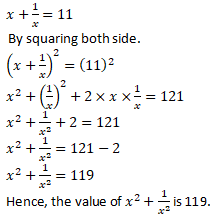Question 5:   If x – 1/x=-1, find the value of x2+1/x2
Solution: 5
It is given that,Exercise 4.2

Question 1: Write the following in the expanded form:
(i) (a+2b+c)2
(ii) (2a-3b-c)2
(iii) (-3x+y+z)2
(iv) (m+2n-5p)2
(v) (2+x-2y)2
(vi) (a2+b2+c2 )2
(vii) (ab+bc+ca)2
(viii) (x/y+y/z+z/x )2
(ix) (a/bc+ b/ac+ c/ab )2
(x) (x+2y+4z)2
(xi) (2x-y+z)2
(xii) (-2x+3y+2z)2
Solution: 1
(i) (a+2b+c)2
By using identity (x + y + z)2 =x2+y2+z2+2xy+2yz+2xz
= a2+(2b)2  +c2+2a×2b+2ac+2×2b×c
= a2+ 4b2+ c2+4ab+2ac+4bc
Hence, the expended form of (a+2b+c)2 is  a2+ 4b2+ c2+4ab+2ac+4bc.

(ii) (2a-3b-c)2
By using identity (x + y + z)2 =x2+y2+z2+2xy+2yz+2xz
= [(2a) + (-3b) + (-c)]2
= (2a)2  +(-3b)2  +(-c)2+2(2a)(-3b)+2(-3b)(-c)+2(2a)(-c)
= 4a2+9b2+c2-12ab + 6bc - 4ca
Hence, the expended form of (2a - 3b - c)2 is 4a2+9b2+c2-12ab + 6bc - 4ca.

(iii) (-3x+y+z)2
By using identity (x + y + z)2 =x2+y2+z2+2xy+2yz+2xz
= (-3x)2+y2+ z2+2(-3x)y + 2yz + 2(-3x)z
= 9x2+y2+z2- 6xy + 2yz -6xz
Hence, the expended form of (-3x+y+z)2 is 9x2+y2+z2- 6xy + 2yz -6xz

(iv) (m+2n-5p)2
By using identity (x + y + z)2 =x2+y2+z2+2xy+2yz+2xz
= m2+(2n) +(-5p)2  + 2m × 2n + (2×2n×-5p) + 2m × -5p
= m2+ 4n2+ 25p2+ 4mn - 20np - 10pm
Hence, the expended form of (m+2n-5p)2  is  m2+ 4n2+ 25p2+ 4mn - 20np - 10pm.

(v) (2+x-2y)2
By using identity (x + y + z)2 =x2+y2+z2+2xy+2yz+2xz
= 22+ x2+ (-2y)2  + 2(2)(x) + 2(x)(-2y) + 2(2)(-2y)
= 4 + x2+ 4y2+ 4 x - 4xy - 8y
Hence, the expended form of (2+x-2y)2 is 4+x2+4y2+4x-4xy-8y.

(vi) (a2+b2+c2)2
By using identity (x + y + z)2 =x2+y2+z2+2xy+2yz+2xz
= (a2)2  +(b2)2  +(c2)2  +2a2b2+2b2c2+2a2c2
= a4+b4+c4+2a2b2+2b2c2+ 2c a2
Hence, the expended form of (a2+b2+c2)2 is a4+b4+c4+2a2 b2+2b2 c2+2c2 a2.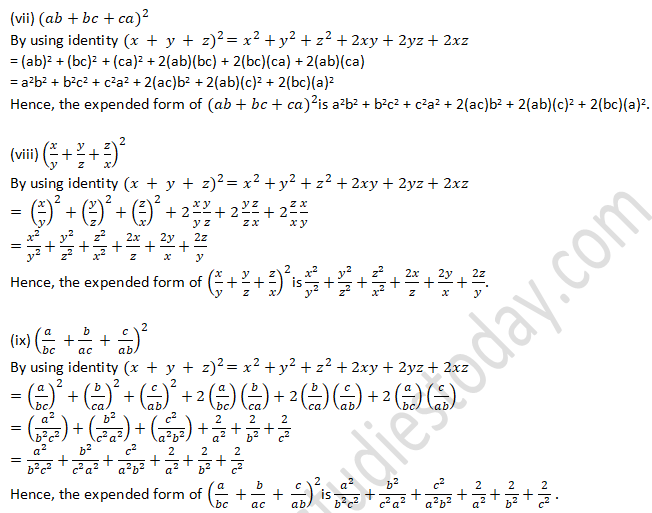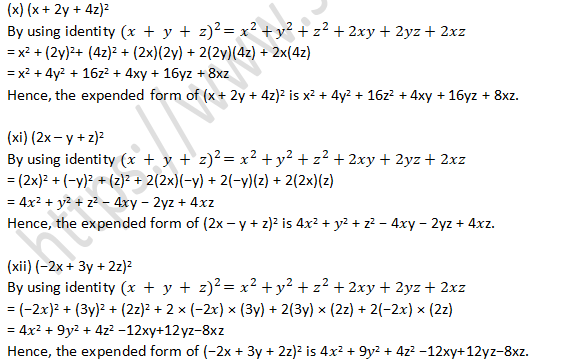Question 2:  Simplify
(i) (a + b + c)2 + (a − b + c)2
(ii) (a + b + c)2 − (a − b + c)2
(iii) (a + b + c)2 + (a – b + c)2 + (a + b − c)2
(iv) (2x + p − c)2 − (2x − p + c)2
(v) (x2 + y2 − z2)2 − (x2 − y2 + z2)2
Solution: 2
(i) (a + b + c)2 + (a − b + c)2
By using identity (x + y + z)2 =x2+y2+z2+2xy+2yz+2xz
= (a2 + b2 + c2 + 2ab+2bc+2ca) + (a2 + (−b)2 + c2 −2ab−2bc+2ca)
= 2a2 + 2b2 + 2c2 + 4ca
Hence, the simplest form of (a + b + c)2 + (a − b + c)2 is 2a2 + 2b2 + 2c2 + 4ca.

(ii) (a + b + c)2 − (a − b + c)2
By using identity (x + y + z)2 =x2+y2+z2+2xy+2yz+2xz
= (a2 + b2 + c2 + 2ab + 2bc + 2ca) − (a2 + (−b)2 + c2 −2ab − 2bc + 2ca)
= a2 + b2 + c2 + 2ab + 2bc + 2ca − a2 − b2 − c2 + 2ab + 2bc − 2ca
= 4ab + 4bc
Hence, the simplest form of (a + b + c)2 − (a − b + c)2 is 4ab + 4bc.

(iii) (a + b + c)2 + (a – b + c)2 + (a + b − c)2
By using identity (x + y + z)2 =x2+y2+z2+2xy+2yz+2xz
= a2 + b2 + c2 + 2ab + 2bc + 2ca + (a2 + b2 + c)2 − 2ab − 2cb + 2ca) + (a2 + b2 + c2 + 2ab − 2bc – 2ca)
= 3a2 + 3b2 + 3c2 + 2ab − 2bc + 2ca
Hence, the simplest form of (a + b + c)2 + (a – b + c)2 + (a + b − c)2 is 3a2 + 3b2 + 3c2 + 2ab − 2bc + 2ca.

(iv) (2x + p − c)2 − (2x − p + c)2
By using identity (x + y + z)2 =x2+y2+z2+2xy+2yz+2xz
= [(2x)2+p2+(-c)2+2×2x×p+2×p×-c+2×2x×-c]-[(-2x)2+p2+c2+2×-2x×p+2×p×c+2×-2x×c]
= [4x2+p2+c2+4xp-2pc-4cx] − [4x2+p2+ c2- 4xp-2pc+4cx]
= 4x2+p2+c2+4xp-2pc-4cx-4x2-p2- c2+ 4xp + 2pc- 4cx
= 8xp - 8xc
=8(xp - xc)
Hence, the simplest form of (2x + p − c)2 − (2x − p + c)2 is 8(xp - xc).

(v) (x2 + y2 − z2)2 − (x2 − y2 + z2)2
By using identity  (x + y + z)2 =x2+y2+z2+2xy+2yz+2xz
= (x2 + y2 + (−z)2)2 − (x2 − y2 + z2)2
= [x4 + y4 + z4 + 2x2y2 – 2y2z2 – 2x2z2 − [x4 + y4 + z4 − 2x2y2 − 2y2z2 + 2x2z2]
= 4x2y2 – 4z2x2
Hence, the simplest form of (x2 + y2 − z2)2 − (x2 − y2 + z2)2 is 4x2y2 – 4z2x2.

Question 3: If a + b + c = 0 and a2 + b2 + c2 = 16, find the value of ab + bc + ca.
Solution: 3
It is given that,
a + b + c = 0
a2 + b2 + c2 = 16
By squaring both sides,
(a + b + c)2 = (0)2
By using identity of (a + b + c)2 = a2 + b2 + c2 + 2(ab + bc + ca)
a2 + b2 + c2 + 2(ab + bc + ca) = 0
16 + 2(ab + bc + c) = 0
2(ab+bc+ca)=-16
ab+bc+ca=-16/2
ab + bc + ca= -8
Hence, the value of  ab + bc + ca is -8

Exercise 4.3

Question 1: Find the cube of each of the following binomial expressions:
(i) (1/x + y/3)
(ii) (3/x  – 2/x2 )
(iii) (2x + 3/x)
(iv) (4 – 1/3x)
Solution:Question 2: Simplify each of the following: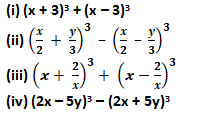Solution:Question 3:   If a + b = 10 and ab = 21, find the value of a3 + b3.
Solution:
It is given that,
a + b = 10
ab = 21
From a + b = 10
By the Cubing both sides, we get
(a + b)3 = (10)3
By the identity of (a + b)3 = a3 + b3 + 3ab (a + b)
a3 + b3 + 3ab (a + b) = 1000
a3 + b3 + 3 x 21 x 10 = 1000
a3 + b3 + 630 = 1000
a3 + b3 = 1000 – 630 = 370
a3 + b3 = 370
Hence, the value of a3 + b3 is 370.

Question 4: If a – b = 4 and ab = 21, find the value of a3 – b3.
Solution:
It is given that,
a – b = 4…………….(i)
ab = 21……………..(ii)
From Equation (i)
a – b = 4
By the Cubing both sides, we get
(a – b)3 = (4)3
By the identity of (a + b)3 = a3 + b3 + 3ab (a + b)
a3 – b3 – 3ab (a – b) = 64
a3 – b3 – 3 × 21 x 4 = 64
a3 – b3 – 252 = 64
a3 – b3 = 64 + 252
a3 – b3 = 316
Hence, the value of a3 – b3 is 316

Question 5: If x +  1/x= 5, find the value of x3+ 1/x3  .
Solution:
We have,Question 6:  If x –  1/x= 7, find the value of x3  –  1/x3  .
Solution:
We have,
x –  1/x  = 7Question 7: If x –  1/x  = 5, find the value of x3  –  1/x3  .
Solution:
We have,
x –  1/x= 5Question 8:  If (x2  +  1/x2 ) = 51, find the value of  .
Solution:
It is given that,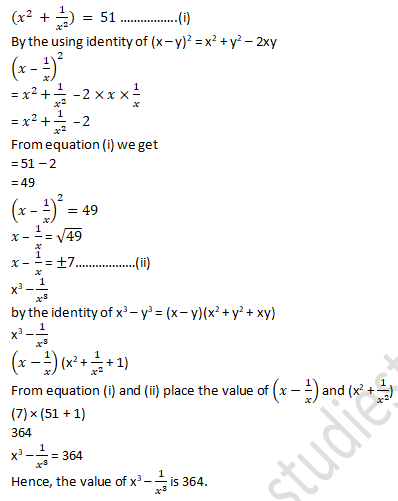Question 9: If (x2+1/x2 )= 98, find the value of (x3+1/x3 )
Solution:
It is given that,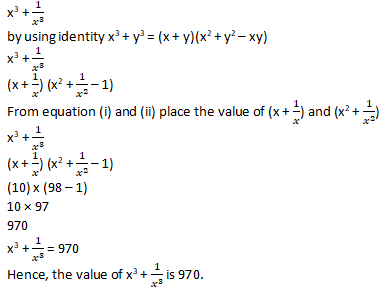Question 10: If 2x + 3y = 13 and xy = 6, find the value of 8x3 + 27y3.
Solution:
It is given that,
2x+3y=13 and xy=6
By cubing both the sides, we get
(2x + 3y)3 = (13)3
By the identity of (a + b)3 = a3 + b3 + 3ab (a + b)
(2x)3 + (3y)3 + 3 × (2x)(3y) (2x + 3y) = 13 × 13 × 13
8x3 + 27y3 + 18xy (2x + 3y) = 2197
8x3 + 27y3 + 18 x 6 x 13 = 2197
8x3 + 27y3 + 1404 = 2197
8x3 + 27y3 = 2197 – 1404
8x3 + 27y3 = 793
Hence, the value of 8x3 + 27y3 is 793.

Question 11: If 3x – 2y= 11 and xy = 12, find the value of 27x3 – 8y3.
Solution:
It is given that
3x – 2y = 11 and xy = 12
By cubing both the sides, we get
(3x – 2y)3 = (11)3
By the identity of (a - b)3 = a3 - b3 - 3ab (a - b)
(3x)3 – (2y)3 – 3 (3x)( 2y) (3x – 2y) = 1331
27x3 – 8y3 – 18xy (3x -2y) = 1331
27x3 – 8y3 – 18 x 12 x 11 = 1331
27x3 – 8y3 – 2376 = 1331
27x3 – 8y3 = 1331 + 2376
27x3 – 8y3 = 3707
Hence, the value of 27x3 – 8y3 is 3707.

Exercise 4.4

Question 1:  Find the following products:
(i) (3x + 2y)(9x2 – 6xy + 4y2)
(ii) (4x – 5y)(16x2 + 20xy + 25y2)
(iii) (7p4 + q)(49p8 – 7p4q + q2)
(iv) (x/2+ 2y) (x2/4– xy + 4y2
(v) (3/x – 5/y)(9/x2  + 25/y2  + 15/xy)
(vi) (3 + 5/x)(9 – 15/x + 25/x2)
(vii) (2/x + 3x)(4/x2  + 9x2 – 6)
(viii) (3/x – 2x2)(9/x2  + 4x4 – 6x)
(ix) (1 – x)(1 + x + x2)
(x) (1 + x)(1 – x + x2)
(xi) (x2 – 1)(x4 + x2 +1)
(xii) (x3 + 1)(x6 – x3 + 1)
Solution:
(i) (3x + 2y)(9x2 – 6xy + 4y2)
= (3x + 2y)[(3x)2 – (3x)(2y) + (2y)2]
By using identity of a3 + b3 = (a + b)(a2 + b2 – ab)
Here a = 3x and b = 2y
= (3x)3 + (2y)3
= 27x3 + 8y3
Hence, the product of (3x + 2y)(9x2 – 6xy + 4y2) is  27x3 + 8y3.

(ii) (4x – 5y)(16x2 + 20xy + 25y2)
= (4x – 5y)[(4x)2 + (4x)(5y) + (5y)2)]
By using identity of a3 – b3 = (a – b)(a2 + b2 + ab)
Here a = 4x and b = 5y
= (4x)3 – (5y)3
= 64x3 – 125y3
Hence, the product of (4x – 5y)(16x2 + 20xy + 25y2) is 64x3 – 125y3.

(iii) (7p4 + q)(49p8 – 7p4q + q2)
= (7p4 + q)[(7p4)2 – (7p4)(q) + (q)2)]
By using identity of a3 + b3 = (a + b)(a2 + b2 – ab)
Here a=7p2 and b=q
= (7p4)3 + (q)3
= 343 p12 + q3
Hence, the product of (7p4 + q)(49p8 – 7p4q + q2) is 343 p12 + q3.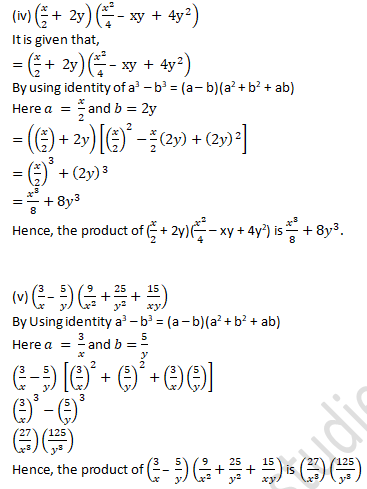(ix) (1 – x)(1 + x + x2)
By Using identity a3 – b3 = (a – b)(a2 + b2 + ab)
(1 – x)(1 + x + x2)
(1 – x)[(12 + (1)(x)+ x2)]
(1)3 – (x)3
1 – x3
Hence, the product of (1 – x)(1 + x + x2) is 1 – x3.

(x) (1 + x)(1 – x + x2)
By Using identity a3 + b3 = (a + b)(a2 + b2 – ab)
(1 + x)(1 – x + x2)
(1 + x)[(12 – (1)(x) + x2)]
(1)3 + (x)3
1 + x3
Hence, the product of (1 + x)(1 – x + x2) is 1 + x3

(xi) (x2 – 1)(x4 + x2 +1)
By Using identity a3 – b3 = (a – b)(a2 + b2 + ab)
(x2 – 1)[(x2)2 – (1)2 + (x2)(1)]
(x2 – 1)[(x2)2 – (1)2 + x2]
(x2)3 – (1)3
x6 – 1
Hence, the product of (x2 – 1)(x4 + x2 +1) is x6 – 1.

(xii) (x3 + 1)(x6 – x3 + 1)
By Using identity a3 + b3 = (a + b)(a2 + b2 – ab)
(x3 + 1)[(x3)2 – (x3)(1) + 12]
(x3 + 1)[(x3)2 – x3 + 12]
(x3)3 + 13
x9 + 1
Hence, the product of (x3 + 1)(x6 – x3 + 1) is x9 + 1.

Question 2: If x = 3 and y = -1, find the values of each of the following using in identity:
(i) (9y2 – 4x2)(81y4 + 36x2y2 + 16x4)
(ii) (3/x – x/3)(x2/9 + 9/x2  + 1)
(iii) (x/7 + y/3)(x2/49 + y2/9 – xy/21)
(iv) (x/4 – y/3)(x2/16 + xy/12 + y2/9)

(v) (5/x + 5x)(25/x2  – 25 + 25x2)
Solution:
(i) It is given that,
(9y2 – 4x2)(81y4 + 36x2y2 + 16x4)
(9y2 – 4x2) [(9y2)2 + 9y2 x 4x2 + (4x2)2
By using identity of a3 + b3 = (a + b)(a2 + b2 – ab)
(9y2)3 – (4x2)3
729y6 – 64x6
It is given that the value of x = 3 and y = -1
Value of x and y put in above equation
729(-1)6 – 64(3)6
729 – 46656
– 45927
Hence, the Value of (9y2 – 4x2)(81y4 + 36x2y2 + 16x4) is - 45927.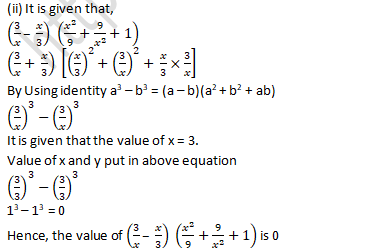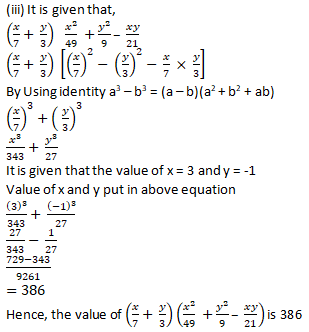Question 3:  If a + b = 10 and ab = 16, find the value of a2 – ab + b2 and a2 + ab + b2.
Solution:
It is given that,
a + b = 10 …………………..(i)
ab = 16 …………………..(ii)
By squaring both the sides of Equation (i)
(a + b)2 = (10)2
By using identity (a + b)2 = a2 + b2 + 2ab
a2 + b2 + 2ab = 100
The value of ab is 16
a2 + b2 + 2 x 16 = 100
a2 + b2 + 32 = 100
a2 + b2 = 100 – 32 = 68
a2 + b2 = 68
So the value of a2  – ab + b2 is
a2 + b2 – ab
= 68 – 16
= 52
And the value of a2 + ab + b2
a2 + b2 + ab
= 68 + 16
= 84
Hence, the value of a2  – ab + b2 is 52 and a2 + ab + b2 is 84.

Question 4: If a + b = 8 and ab = 6, find the value of a3 + b3.
Solution:
It is given that,
a + b = 8……………………(i)
ab = 6………………….(ii)
By cubing both the sides of Equation (i)
(a + b)3 = (8)3
By Using identity (a + b)3 = a3 + b3 + 3ab (a + b)
a3 + b3 + 3ab (a + b) = 512
It is given that the value of a + b = 8 and ab = 6
Value of x and y put in above equation
a3 + b3 + 3 x 6 x 8 = 512
a3 + b3 + 144 = 512
a3 + b3 = 512 – 144 = 368
a3 + b3 = 368
Hence, the value of a3 + b3 is 368.
Exercise 4.5

Question 1: Find the following products:
(i) (3x + 2y + 2z) (9x2 + 4y2 + 4z2 – 6xy – 4yz – 6zx)
(ii) (4x – 3y + 2z) (16x2 + 9y2 + 4z2 + 12xy + 6yz – 8zx)
(iii) (2a – 3b – 2c) (4a2 + 9b2 + 4c2 + 6ab – 6bc + 4ca)
(iv) (3x -4y + 5z) (9x2 + 16y2 + 25z2 + 12xy- 15zx + 20yz)
Solution:
(i) It is given that,
(3x + 2y + 2z) (9x2 + 4y2 + 4z2 – 6xy – 4yz – 6zx)
(3x+ 2y + 2z) [(3x)2 + (2y)2 + (2z)2 – 3x x 2y – 2y x 2z – 2z x 3x]
By using identity of a3 + b3 + c3 - 3abc = (a + b + c)(a2 + b2 + c2 - ab - bc - ca)
(3x)3 + (2y)3 + (2z)3 – 3 x 3x x 2y x 2z
27x3 + 8y3 + 8z3 – 36xyz
Hence, the value of (3x + 2y + 2z) (9x2 + 4y2 + 4z2 –6xy–4yz –6zx) is 27x3 + 8y3 + 8z3 – 36xyz

(ii) It is given that,
(4x – 3y + 2z) (16x2 + 9y2 + 4z2 + 12xy + 6yz – 8zx)
(4x -3y + 2z) [(4x)2 + (-3y)2 + (2z)2 – 4x x (-3y) – (-3y) x (2z) – (2z x 4x)]
By using identity of a3 + b3 + c3 - 3abc = (a + b + c)(a2 + b2 + c2 - ab - bc - ca)
(4x)3 + (-3y)3 + (2z)3 – 3 x 4x x (-3y) x (2z)
64x3 – 27y3 + 8z3 + 72xyz
Hence, the value of (4x – 3y + 2z) (16x2 + 9y2 + 4z2 + 12xy + 6yz – 8zx) is 64x3 – 27y3 + 8z3 + 72xyz.

(iii) It is given that,
(2a – 3b – 2c) (4a2 + 9b2 + 4c2 + 6ab – 6bc + 4ca)
(2a -3b- 2c) [(2a)2 + (-3b)2 + (-2c)2 – 2a x (-3b) – (-3b) x (-2c) – (-2c) x 2a]
By using identity of a3 + b3 + c3 - 3abc = (a + b + c)(a2 + b2 + c2 - ab - bc - ca)
(2a)3 + (-3b)3 + (-2c)3 -3x 2a x (-3 b) (-2c)
8a3 – 21b3 – 8c3 – 36abc
Hence, the value of (2a -3b- 2c) (4a2 + 9b2 + 4c2 + 6ab – 6bc + 4ca) is 8a3 – 21b3 – 8c3 – 36abc.

(iv) It is given that,
((3x -4y + 5z) (9x2 + 16y2 + 25z2 + 12xy- 15zx + 20yz)
[3x + (-4y) + 5z] [(3x)2 + (-4y)2 + (5z)2 – 3x x (-4y) -(-4y) (5z) – 5z x 3x]
By using identity of a3 + b3 + c3 - 3abc = (a + b + c)(a2 + b2 + c2 - ab - bc - ca)
(3x)3 + (-4y)3 + (5z)3 – 3 x 3x x (-4y) (5z)
27x3 – 64y3 + 125z3 + 180xyz
Hence, the value of (3x – 4y + 5z) (9x2 + 16y2 + 25z2 + 12xy – 15zx + 20yz) is 27x3 – 64y3 + 125z3 + 180xyz.

Question 2: If x + y + z = 8 and xy + yz+ zx = 20, find the value of x3 + y3 + z3 – 3xyz.
Solution:
It is given that,
x+y+z=8………………(i)
xy+yz+zx=20………………….(ii)
By squaring both the sides of Equation (i)
By using identity of (a+b+c)= a2+b2+c2+2(ab+bc+ca)
(x + y + z)2 = (8)2
x2 + y2 + z2 + 2(xy + yz + zx) = 64
x2 + y2 + z2 + 2 x 20 = 64
x2 + y2 + z2 + 40 = 64
x2 + y2 + z2 = 24
By using identity of a3 + b3 + c3 - 3abc = (a + b + c)(a2 + b2 + c2 - ab - bc - ca)
x3 + y3 + z3 – 3xyz
(x + y + z) [x2 + y2 + z2 – (xy + yz + zx)]
8(24 – 20)
8 x 4
32
Hence, the value of x3 + y3 + z3 – 3xyz is 32.

Question 3: If a +b + c = 9 and ab + bc + ca = 26, find the value of a3 + b3 + c3 – 3abc.
Solution:
It is given that,
a + b + c = 9……………..(i)
ab + bc + ca = 26……………..(ii)
Squaring, a + b + c = 9 both sides, we get
By squaring both the sides of Equation (i)
By using identity of (a+b+c)= a2+b2+c2+2(ab+bc+ca)
(a + b + c)2 = (9)2
a2 + b2 + c2 + 2 (ab + bc + ca) = 81
a2 + b2 + c2 + 2 x 26 = 81
a2 + b2 + c2 + 52 = 81
a2 + b2 + c2 = 29
By using identity of a3 + b3 + c3 - 3abc = (a + b + c)(a2 + b2 + c2 - ab - bc - ca)a3 + b3 + c3 - 3abc
(a + b + c)(a2 + b2 + c2 - ab - bc - ca) ………………..(iii)
Put the value of a + b + c = 9 and ab + bc + ca = 26 in equation (iii)
9 [29 – 26]
9 x 3
27
Hence, the value of a3 + b3 + c3 – 3abc is 27

Exercise VSAQs..........................

Question 1:  If x + 1/x = 3, then find the value of x2 + 1/x2 .
Solution:
It is given that,
x + 1/x = 3
By the squaring both sides,
(x+1/x)2 = (3)2
By the identity of (a + b)2 = a2 + 2ab + b2
x2+1/x2 + 2x×1/x=9
x2+1/x2 + 2=9
x2+1/x2 =9-2
x2+1/x2 =7
Hence, the value of x2+1/x2  is 7.

Question 2:  If x + 1/x = 3, then find the value of x6 + 1/x6 .
Solution:
It is given that,
x + 1/x = 3
By the squaring both sides,
(x+1/x)2 = (3)2
By the identity of (a + b)2 = a2 + 2ab + b
x2+1/x2 + 2x×1/x=9
x2+1/x2 + 2=9
x2+1/x2 =9-2
x2+1/x2 =7 ………………(i)
By the cubing both sides of equation (i),
(x2+1/x2 )3= (7)3
By the identity of (a + b)3 = a3 +  b3 + 3(a + b)
x6 + 1/x6  + 3 (x2 + 1/x2 ) = 343
x6 + 1/x6 +3 x 7=343
x6 + 1/x6   = 322
Hence, the value of x6 + 1/x6  is 322.

Question 3: If a + b = 7 and ab = 12, find the value of a2 + b2.
Solution:
It is given that,
a + b = 7………………(i)
ab = 12………………(ii)
By the squaring both sides of equation (i),
(a + b)2 = (7)2
By the identity of (a + b)2 = a2 + 2ab + b
a2 + b2 + 2ab = 49
Put the value of ab in above equation,
a2 + b2+ 2 x 12 = 49
a2 + b2 + 24 = 49
a2 + b2 = 25
Hence, the value of a2 + b2 is 25.

Question 4: If a – b = 5 and ab = 12, find the value of a2 + b2.
Solution:
It is given that,
a – b = 5………………(i)
ab = 12………………(ii)
By the squaring both sides of equation (i),
(a – b)2 = (5)2
By the identity of (a - b)2 = a2 - 2ab + b2
a2 + b2 – 2ab = 25
a2 + b2 – 2 x 12 = 25
a2 + b2 – 24 = 25
a2 + b2 = 49
Hence, the value of a2 + b2 is 49.